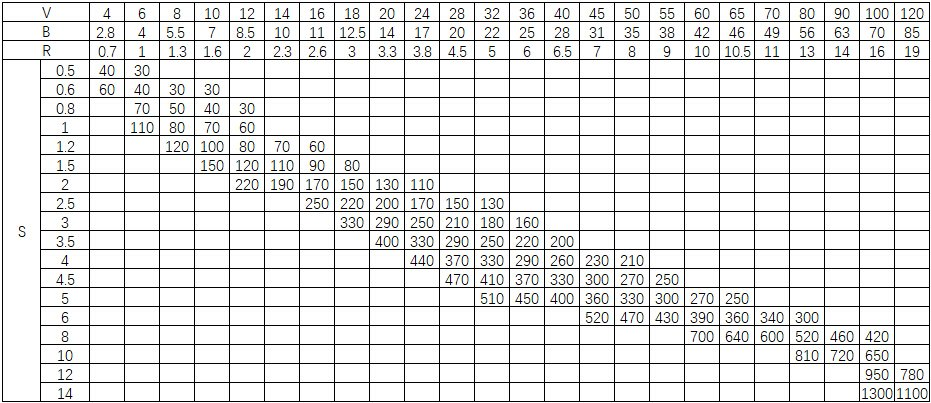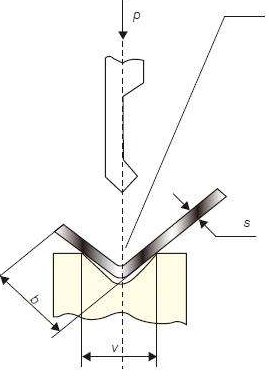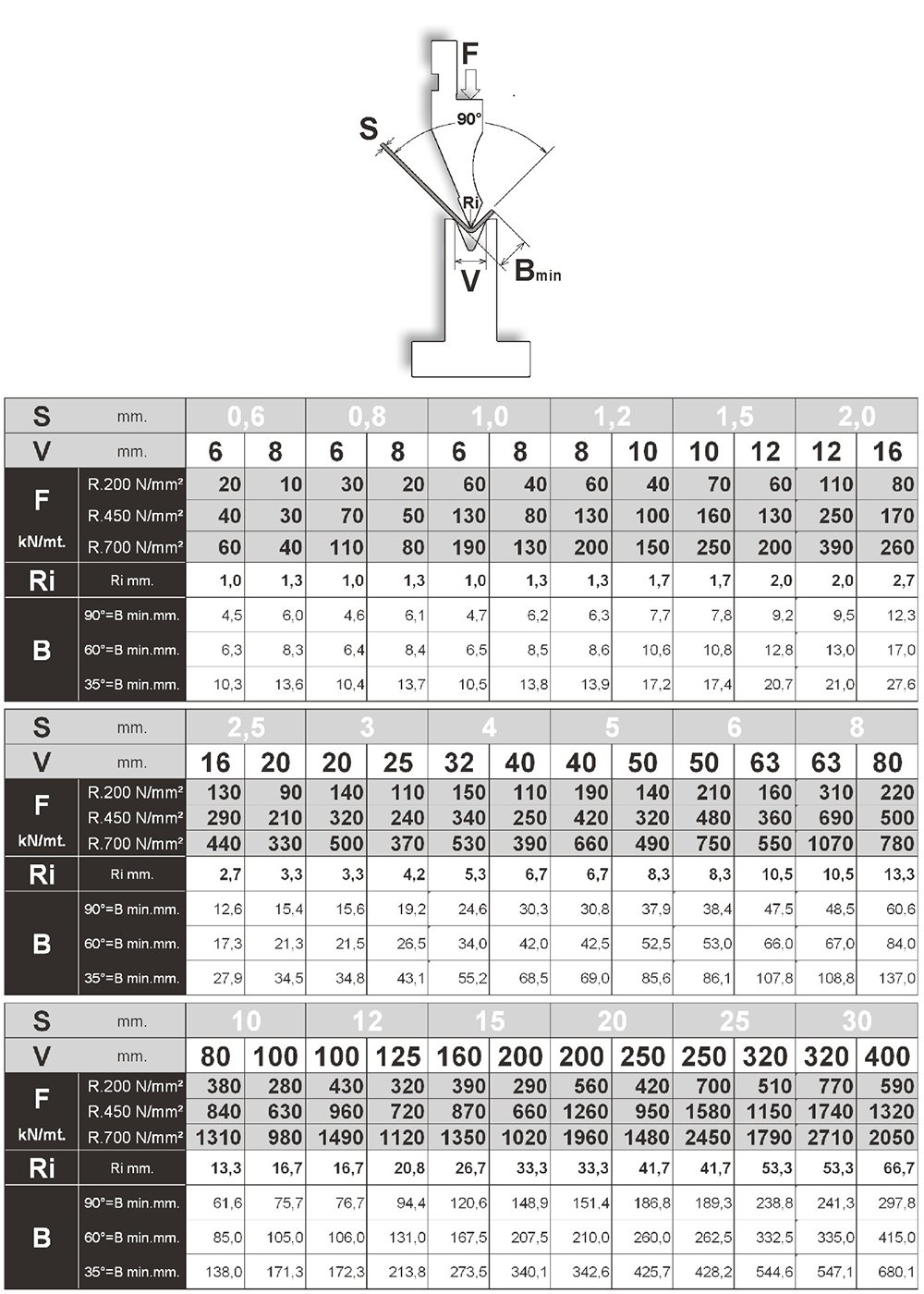# How to Calculate Press Brake Tonnage (By Chart, Formula & Calculator)

For determining the hydraulic bending force or the tonnage of the press brake that is required to bend the sheet metal in a specific thickness, you have totally the following THREE methods:

#1. By the press brake tonnage chart

#2. By the bending force calculation formula

#3. By the press brake tonnage calculator

Only after the tonnage of the press brake confirmed, then you can spend your time to take other factors (press brake components, toolings, controllers, etc) into consideration before making your decision to purchase the press brake.

In this article, we will show you how to determine the required bending force by your sheet metal.

## #1. By The Press Brake Tonnage Chart

The following press brake tonnage chart is used by many press brake manufacturers both in China and abroad countries.

We know that many press brake suppliers are using a different chart, but most of them are similar and the final result will almost the same. So just take one of them as your reference.Note: if there are no special requirements for bending radius, the slot width V should be 8-10 times plate thickness.

The tonnage as shown in the above press brake tonnage chart is calculated based on the sheet metal with the tensile strength σb=450N/mm² and length L=1m.

Now you have the bending force chart, the question is how to find the press brake tonnage in the above chart?

First, you need to know what does “S” “V” “B” “R” means in the above chart. Check out the following pictures you will understand what do they mean.• P = Bending force
• S = Thickness of sheet
• V = Vee opening of the bottom die
• B = Min bending flange

Let’s say the thickness of your metal sheet S=4mm, generally the vee opening of the bottom die is 8 times the thickness of the sheet. However, for the thicker plate, the vee opening should be bigger.

The following recommend vee opening of the bottom die can be your reference.

S 0.5-3mm 3-8mm 9-10mm >12mm
V 6*S 8*S 10*S 12*S

Now we back to the chart, find the S data in the chart which is “4”, and now we know the “V” should be 4*8=32. And you can see that the intersection of the row and column where the “S” and “V” stay is “330”. The unit of “330” is “KN”.

Now we know that for bending the sheet metal with 4mm thickness and 1m length, the required tonnage is 330KN.

If bend the 4mm sheet in 3 meters, then the tonnage should be 330*3=990KN, which equals to 101 Ton.

Here we come to the conclusion: you should choose at least 100ton press brake. However, we recommend you to choose the bigger tonnage like 120Ton because if the press brake works in full load for a long time, the service life of the machine will be shortened.

## #2. By The Bending Force Calculation Formula

Except for the bending chart, you can also use the bending force formula to calculate the required bending force by yourself.### #1 Formula

The press brake bending force calculation formula is: P=650*S²*L/V (σb=450N/mm²)

• P: bending force (KN)
• S: plate thickness (mm)
• L: plate width (m)
• V: bottom die slot width (mm)

For example:

Plate thickness S=4mm, width L=3m, σb=450N/mm²

Generally slot width V=S*8

Therefore P=650*4²*3/4*8=975 (KN) = 99.5 (Ton)

The result is very close to the data in the bending force chart.

As you can see, the method #1 to calculate the press brake tonnage are based on the mild steel material.

What if the material is stainless steel, aluminum or brass?

It’s simple, multiply the results calculated by the above formula by the coefficients in the following table:

Material Coefficients
Mild Steel 1
Stainless Steel 1.6
Aluminum 0.65
Brass 0.5

### #2 Formula

Another press brake tonnage calculation formula is: P=1.42*σb*S²*L/V

• P: bending force (KN)
• S: plate thickness (mm)
• L: plate width (m)
• V: bottom die slot width (mm)
• σb: tensile strength (Mpa)

For example:

Plate thickness S=4mm, width L=3m, σb=450N/mm²

Generally slot width V=S*8

Therefore P=1.42*450*4²*3/4*8=958.5 (KN) = 96 (Ton)

For bending the sheet metal with different materials, the key point is to find out the tensile strength of the specific material, then you will get the required bending force by the above formula.

### New Calculation Formula

In free bending, the opening width V of the lower die selected is 8 to 10 times the sheet thickness S.

And the press brake manufacturers always list the corresponding values of the die width V and the inner diameter r of the bending workpiece on the bending force parameter table.

Generally r=(0.16~0.17)V.

However, when the inner radius r is not (0.16-0.17)V, then the above two calculation formula is not applied any more. Then you should refer to the new calculation method to calculate the necessary bending force or press brake tonnage.

## #3. By The Press Brake Tonnage Calculator

Finally, I strongly suggest you use the following press brake tonnage calculator because this is probably the best and easiest way to calculate the required bending force.

There is one similar press brake bending chart made by one European professional press brake toolings manufacturer. This can also be your reference, below is it:### 8 thoughts on “How to Calculate Press Brake Tonnage (By Chart, Formula & Calculator)”

1. Dear sir if i choose beding radius R15 but thickness of part is 5 mm, then which v opening i have considered and what should be the minimum length of “B”.

1. C channel size is 2780mm/530mm/12mm.
Both side height is 75mm from bottom.And radius at bend is 38mm.
How much ton hydraulic press i can use and how many nos.of identical cylinders required in a row..

2. C channel size is 2780mm/530mm/12mm.C channel to make length wise.Before bending size of plate will be 2780mm/680mm/12mm.
Both side height is 75mm from bottom.And radius at bend is 38mm.
How much ton hydraulic press i can use and how many nos.of identical cylinders required in a row..

3. Great article ! Just one question – #2 in the formula 1.42 figure – where is that coming from ? Many thanks 😉